# 机器学习-感知机perceptron

## 定义

f(x)=sign(wx+b)

i=1mwixi=w1x1+w2x2+...+wnxn

sign为符号函数，即

f(x)={+11if x>=0else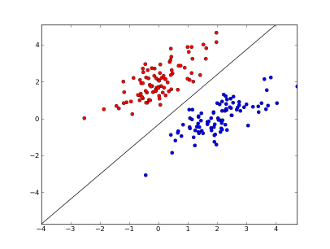## 学习策略

1w|wx0+b|

yi(wxi+b)>0

1wyi(wx0+b)

1wxiϵMyi|wx0+b|

L(w,b)=xiϵMyi(wx0+b)

## 学习算法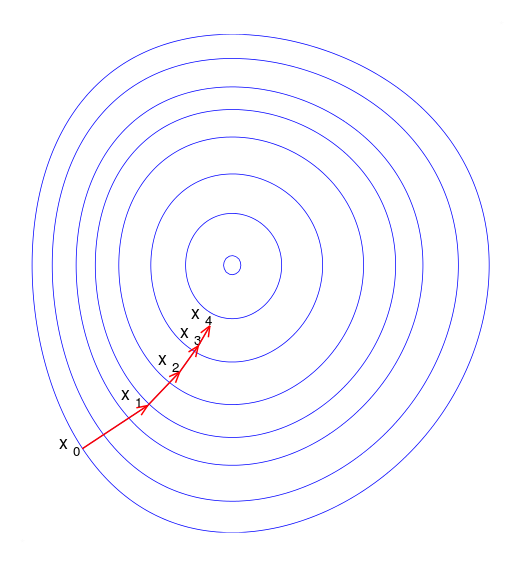minw,bL(w,b)=xiϵMyi(wx0+b)

L(w,b)w=xiϵMyixi

L(w,b)b=xiϵMyi

w:=w+ηyixi

b:=b+ηyi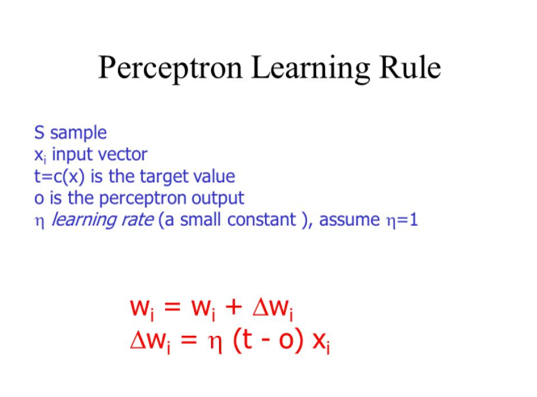输入：T={(x1,y1),(x2,y2)...(xN,yN)}（其中xi∈X=Rn，yi∈Y={-1, +1}，i=1,2...N，学习速率为η）

(1) 初始化w0,b0，权值可以初始化为0或一个很小的随机数
(2) 在训练数据集中选取（x_i, y_i）
(3) 如果yi(w xi+b)≤0
w = w + ηy_ix_i
b = b + ηy_i
(4) 转至（2）,直至训练集中没有误分类点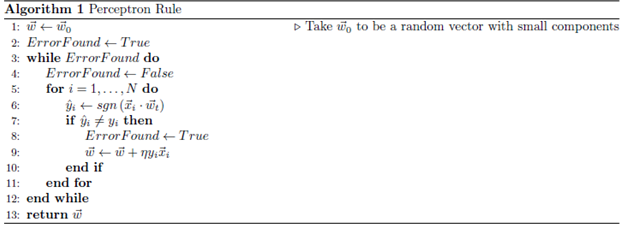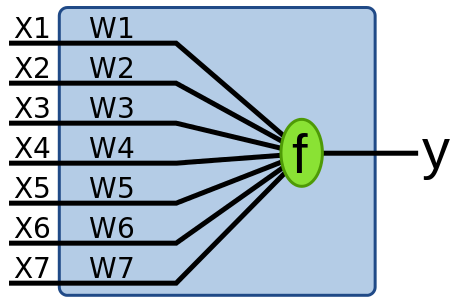yj(t)=f[w(t)xj+b]=f[w1(t)xj,1+w2(t)xj,2++wn(t)xj,n+b]

## 实例

（1） 令 w0=0,b0=0 $w_0=0,b_0=0$
（2） 随机的取一个点，如 x1 $x_1$,计算 y1(w0x1+b0) $y_1(w_0\cdot x_1+b_0)$,结果为0，表示未被正确分类，根据下面的式子更新 w,b $w,b$（此例中，我们将学习率 η $\eta$设置为1）:

ww+ηyixi

bb+ηyi

w1=w0+ηy1x1=(3,3)T

b1=b0+ηy1=1

w1x+b1=3x(1)+3x(2)+1

（3）接着继续，计算各个点是否分错，通过计算得到， x1x2 $x_1和x_2$两个点， yi(w0xi+b1) $y_i(w_0\cdot x_i+b_1)$都大于0，所以是被正确分类的点，无需修改权值w和bias项；而对于 x3 $x_3$通过计算得到 y3(w0x3+b1)<0 $y_3(w_0\cdot x_3+b_1)<0$,误分了，所以修改权值：

w2=w1+y3x3=(2,2)T

b2=b1+y3=0

w2x+b2=2x(1)+2x(2)

……
……
……

w7=(1,1)T,b7=3

f(x)=sign(x(1)+x(2)3)

## 小结

 统计学习方法， 李航 著
 Wikiwand之Perceptron http://www.wikiwand.com/en/Perceptron
 Wikipedia https://en.wikipedia.org/wiki/Machine_learning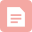博客

01-04327博客

03-165630博客

01-034462博客

01-1957博客

06-193659博客

02-212万+博客

10-262041博客

04-103989博客

11-181531博客

03-134091博客

09-05472博客

05-03524博客

03-09468博客

12-284万+博客

07-237535博客

02-12959

### “相关推荐”对你有帮助么？

•非常没帮助
•没帮助
•一般
•有帮助
•非常有帮助¥2 ¥4 ¥6 ¥10 ¥20余额支付 (余额：-- )扫码支付获取中扫码支付点击重新获取扫码支付1.余额是钱包充值的虚拟货币，按照1:1的比例进行支付金额的抵扣。
2.余额无法直接购买下载，可以购买VIP、C币套餐、付费专栏及课程。余额充值